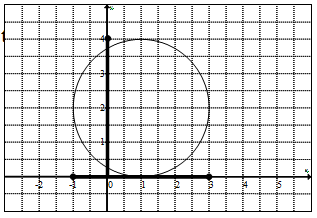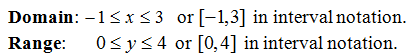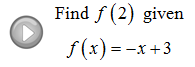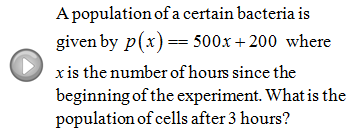## Pages

Showing posts with label range. Show all posts
Showing posts with label range. Show all posts

### Relations, Graphs, and Functions

In this section we will study functions in more depth and begin by defining a relation as any set of ordered pairs.
An example of a relation might look like { (2,3), (2,5), (0,1) }. Here we have a relation consisting of three ordered pairs or points on the Cartesian coordinate system. We are familiar with ordered pairs and usually see them denoted as (x, y).  Typically the x-value (the first component) will be the independent variable or input and the y-value (the second component) is the dependent variable or output.
The example { (2,3), (2,5), (0,1) } is NOT a function because the x-value 2 is assigned more than one y-value, namely 3 and 5. For every x-value there can be only one y-value. Next we define the domain as the set of x-values and the range as the set of y-values for which the relation is defined.
Example:
Tip: When looking at a list of ordered pairs, if there are repeating x-values then the relation is not a function.  This usually indicates that there is an input with multiple outputs. (This does not apply to the y-values)

Determine if the relations are functions.  If so state the domain and range.
Relations can consist of an infinite number of ordered pairs, in which case, making a big list would be impossible. A graph can represent a relation by considering it as a big list of ordered pairs.  Each dot on the graph represents an ordered pair (x, y).
Notice that if we can draw a vertical line that intersects the graph twice we will be able to identify one x-value with two corresponding y-values.  Therefore, it can not be a function.
Alternatively, if any vertical line crosses the graph only once then it does represent a function.

Use the vertical line test to determine whether or not the graph represents a function.
Be prepared to state the domain and range given the graph.  Remember to think of the graph as an infinite set of ordered pairs (x, y).  From the graph, determine the x-values and y-values. First shade in the domain and range then convert to interval notation.
It should be clear, at this point, that the circle shown is not a function.  Nonetheless, we still can determine the domain and range of the relation that it represents.YouTube Video:

---

### Introduction to Functions

You may have noticed that we have been evaluating or "plugging-in" lots of numbers to find the corresponding y-values.  We have been doing this to find points on the graph (x, y).  This process can be streamlined using function notation.
This new notation above is read, "y is equal to f of x".  A function is a rule that uniquely assigns one output to every one input. So, at this point we can think of our non-vertical lines as functions.
The domain of a function is the set of inputs, usually the x-values.  The range of a function is the set of outputs, usually the y-values.
Evaluate the given function.
Find x.
Do not let the function notation discourage you, it takes some time to get used to.  The main thing to remember is that y = f(x); somtimes x is given and sometimes y or f(x) is given.

Graph the given linear function.
Word Problem. Bill has a popular software company which sells copies of its program for \$149.  If the initial start up cost for the company was \$10,000 and it costs \$12 to produce each copy:
a.  Find a cost function C(x) that models this business.
b.  Find a revenue function R(x) that models this business.
c.  Find the profit function P(x) using your functions above.
d.  Find the profit when 1000 programs are produced and sold.
e.  Find the number of programs that must be sold to break even.

Solution:
a. Cost Function - Include all fixed and variable costs of production.
b. Revenue Function - Include all proceeds from sales.
c. Profit Function - Revenue less cost of production.
d. Here x = 1,000 programs produced and sold.
e. Break Even - Occurs when profit is equal to zero.
Video Examples on YouTube: